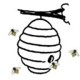## How do I re-arrange…?: Ordering a plot.

One of the most widely seen FAQ coming across list serves and R help sites is the question:

“How do I re-arrange/re-order (plotting geom/aesthetic such as bar/labels) in a (insert plot type here) using(insert graphics system here) in R?”

Don’t believe me? google “reorder factor r plot” and see how many hits you get. I’d venture to say that in almost all cases when you use the words “plot” and “re-arrange”/”re-order” in a question the answer is…

Here’s a quick and dirty R theater demo of how to do this:

``` library(ggplot2)
ggplot(data=mtcars, aes(y=as.factor(carb), x=mpg, colour=hp)) +
geom_point()

# Rearrange_Guy: But I want 2 to come first and 8 last
# Helpful_Gal: OK use rev with levels

mtcars\$carb2 <- factor(mtcars\$carb, levels=rev(levels(factor(mtcars\$carb))))

ggplot(data=mtcars, aes(y=carb2, x=mpg, colour=hp)) +
geom_point()

# Rearrange_Guy: Well I just want to specify the order
# Helpful_Gal: OK type it in by hand then

mtcars\$carb2 <- factor(mtcars\$carb, levels=c("1", "2", "3", "6", "8", "4"))
ggplot(data=mtcars, aes(y=carb2, x=mpg, colour=hp)) +
geom_point()

# Rearrange_Guy: What about faceting?  I bet it doesn't work for that.
# Helpful_Gal: Um yes it does.

ggplot(data=mtcars, aes(y=carb2, x=mpg, colour=hp)) +
geom_point() + facet_grid(cyl~.)

# Rearrange_Guy: OK Helpful_Gal I want it to go 6, 4, and then 8

mtcars\$cyl2 <- factor(mtcars\$cyl, levels=c("6", "4", "8"))
ggplot(data=mtcars, aes(y=carb2, x=mpg, colour=hp)) +
geom_point() + facet_grid(cyl2~.)

# Rearrange_Guy: Why do you keep making new variables?
# Helpful_Gal: It's probably not the best idea to overwrite variables just for the sake of plotting
# Rearrange_Guy: Thank you for showing me the way of re-ordering and re-arranging.

So if you catch yourself using “re-arrange”/”re-order” and “plot” in a question think…

factor & levelsData Scientist, open-source developer , #rstats enthusiast, #dataviz geek, and #nlp buff
This entry was posted in factor, ggplot2 and tagged , , , , , , , , , , , . Bookmark the permalink.

### 7 Responses to How do I re-arrange…?: Ordering a plot.

1.g2-f05a42a0b8a5a1a90999bf19a2b46661 says:

Really great post. I’m totally linking this next time I see one of these posts on the ggplot2 mailing list.

2.marianina says:

That was great help, thanks!

3.Aidan says:

This will work for barplot() too won’t it? Only the levels command is sorting the axis labels but not the data so my graph looks like:
¦A¦_¦B¦_¦C¦_¦D¦
A D B D